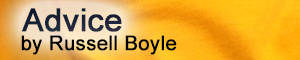The number 15 is 16 minus 1, or 2 to the power 4 less 2 to the power zero 2 is the first prime The prime factors of 15 are 3 and 5 The sum of these factors is 8 8 is 2 cubed The sum of the digits in 15 is 6 6 is a perfect number The absolute difference of the digits is 4 4 is a perfect square The difference between perfect number 6 and perfect square 4, is 2 2 is the only even prime So, after all of this, here are 15 words of advice for success in mathematics and life Go deep and broad Aim high, not low Be honest, sincere and patient And persevere Copyright © Russell BoyleOrder an anthology Testimonials Home page